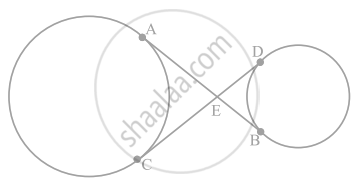# In figure, common tangents AB and CD to two circles intersect at E. Prove that AB = CD. - Mathematics

Sum

In figure, common tangents AB and CD to two circles intersect at E. Prove that AB = CD.#### Solution

Given: AB and CD are two tangents to two circles which intersects at E.

To Prove: AB = CD

Proof:

As

AE = CE   ......[Tangents drawn from an external point to a circle are equal] 

And

EB = ED   ......[Tangents drawn from an external point to a circle are equal] 

AE + EB = CE + ED

AB = CD

Hence Proved!

Concept: Number of Tangents from a Point on a Circle
Is there an error in this question or solution?

#### APPEARS IN

NCERT Mathematics Exemplar Class 10
Chapter 9 Circles
Exercise 9.3 | Q 7 | Page 107
Share